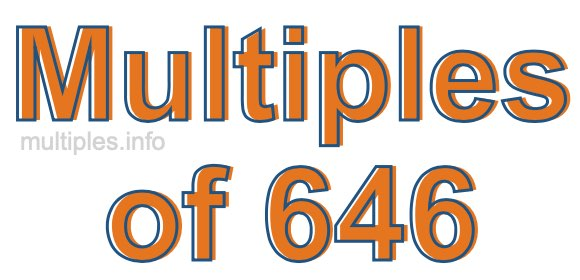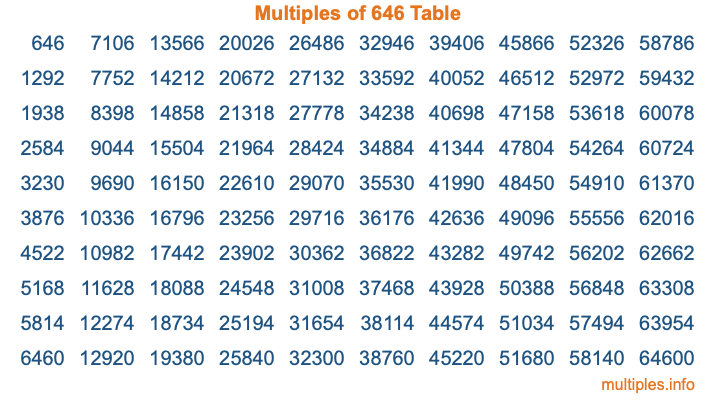Multiples of 646Welcome to the Multiples of 646 page. Here we will first teach you everything you will ever need to know about the multiples of 646, and then give you a study guide summary of everything we taught you to make sure you remember it all. Use this page to look up facts and learn information about the multiples of 646. This page will make you a multiples of six hundred forty-six expert!

Definition of Multiples of 646
Multiples of 646 are all the numbers that when divided by 646 equal an integer. Each of the multiples of 646 are called a multiple. A multiple of 646 is created by multiplying 646 by an integer.

Therefore, to create a list of multiples of 646, you start with 1 multiplied by 646, then 2 multiplied by 646, then 3 multiplied by 646, and so on for as long as you want. Thus, the list of the first five multiples of 646 is 646, 1292, 1938, 2584, and 3230. To see a larger list of multiples of 646, see the printable image of Multiples of 646 further down on this page. We also have a category where you can choose any nth multiple of 646.

Multiples of 646 Checker
The Multiples of 646 Checker below checks to see if any number of your choice is a multiple of 646. In other words, it checks to see if there is any number (integer) that when multiplied by 646 will equal your number. To do that, we divide your number by 646. If the the quotient is an integer, then your number is a multiple of 646.

Is  a multiple of 646?

Least Common Multiple of 646 and ...
A Least Common Multiple (LCM) is the lowest multiple that two or more numbers have in common. This is also called the smallest common multiple or lowest common multiple and is useful to know when you are adding our subtracting fractions. Enter one or more numbers below (646 is already entered) to find the LCM.

Check out our LCM Calculator if you need more details about the Least Common Multiple or if you need the LCM for different numbers for adding and subtraction fractions.

nth Multiple of 646
As we stated above, 646 is the first multiple of 646, 1292 is the second multiple of 646, 1938 is the third multiple of 646, and so on. Enter a number below to find the nth multiple of 646.

th multiple of 646

Multiples of 646 vs Factors of 646
646 is a multiple of 646 and a factor of 646, but that is where the similarities end. All postive multiples of 646 are 646 or greater than 646. All positive factors of 646 are 646 or less than 646.

Below is the beginning list of multiples of 646 and the factors of 646 so you can compare:

Multiples of 646: 646, 1292, 1938, 2584, 3230, etc.

Factors of 646: 1, 2, 17, 19, 34, 38, 323, 646

As you can see, the multiples of 646 are all the numbers that you can divide by 646 to get a whole number. The factors of 646, on the other hand, are all the whole numbers that you can multiply by another whole number to get 646.

It's also interesting to note that if a number (x) is a factor of 646, then 646 will also be a multiple of that number (x).

Multiples of 646 vs Divisors of 646
The divisors of 646 are all the integers that 646 can be divided by evenly. Below is a list of the divisors of 646.

Divisors of 646: 1, 2, 17, 19, 34, 38, 323, 646

The interesting thing to note here is that if you take any multiple of 646 and divide it by a divisor of 646, you will see that the quotient is an integer.

Multiples of 646 Table
Below is an image of the first 100 multiples of 646 in a table. The table is in chronological order, column by column. The first column has the first ten multiples of 646, the second column has the next ten multiples of 646, and so on.The Multiples of 646 Table is also referred to as the 646 Times Table or Times Table of 646. You are welcome to print out our table for your studies.

Negative Multiples of 646
Although not often discussed or needed in math, it is worth mentioning that you can make a list of negative multiples of 646 by multiplying 646 by -1, then by -2, then by -3, and so on, to get the following list of negative multiples of 646:

-646, -1292, -1938, -2584, -3230, etc.

Multiples of 646 Summary
Below is a summary of important Multiples of 646 facts that we have discussed on this page. To retain the knowledge on this page, we recommend that you read through the summary and explain to yourself or a study partner why they hold true.

There are an infinite number of multiples of 646.

A multiple of 646 divided by 646 will equal a whole number.

646 divided by a factor of 646 equals a divisor of 646.

The nth multiple of 646 is n times 646.

The largest factor of 646 is equal to the first positive multiple of 646.

646 is a multiple of every factor of 646.

646 is a multiple of 646.

A multiple of 646 divided by a divisor of 646 equals an integer.

646 divided by a divisor of 646 equals a factor of 646.

Any integer times 646 will equal a multiple of 646.

Multiples of a Number
Here you can get the multiples of another number, all with the same attention to detail as we did for multiples of 646 on this page.

Multiples of
Multiples of 647
Did you find our page about multiples of six hundred forty-six educational? Do you want more knowledge? Check out the multiples of the next number on our list!